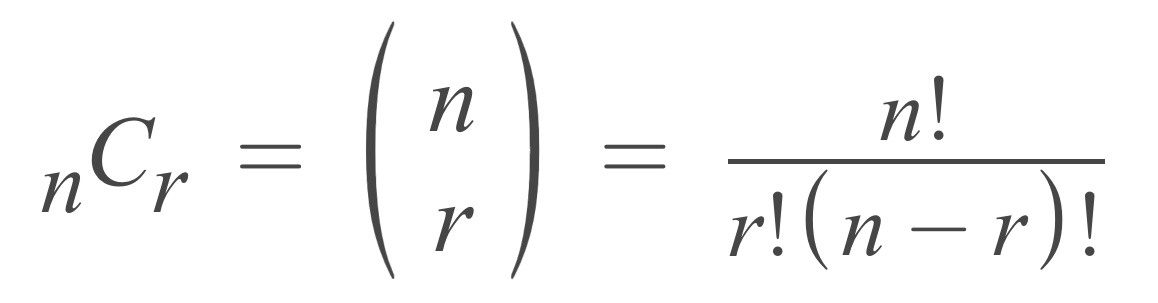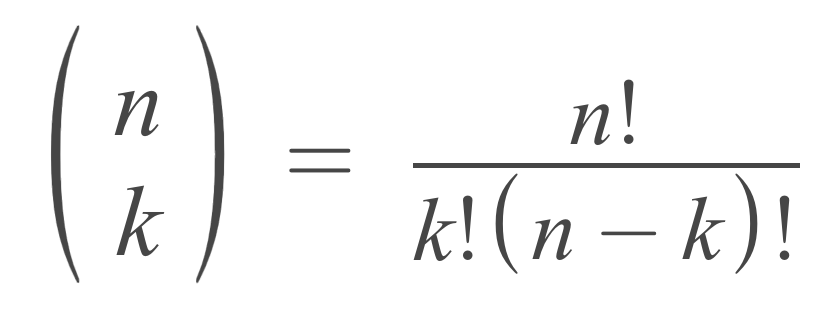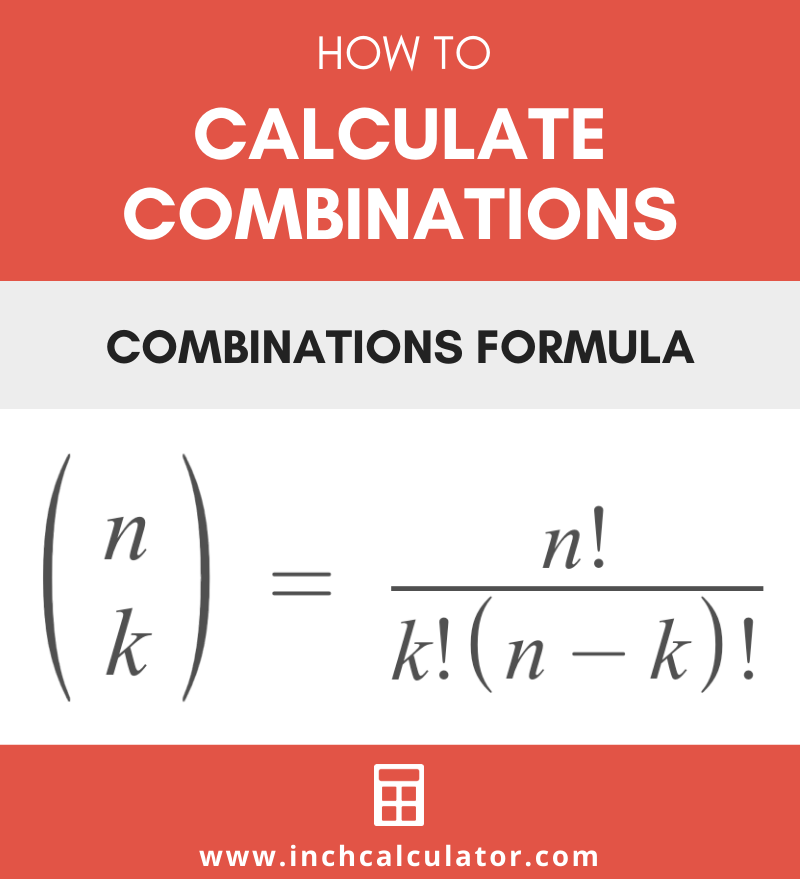# Combination Calculator

Enter the total number of objects and the sample size to calculate the possible combinations with the nCr calculator below.

## Result:

### Number of Permutations P(n,r)

Learn how we calculated this below

## How to Calculate Combinations

Combinations are a set of items from a larger collection without regard to the order of the items in the set.

Lottery tickets are a common example to demonstrate what combinations are. Each ticket represents a combination of numbers from a larger set.

In fact, once you know the total number of combinations, then you can calculate the probability of winning. This is actually the basis for calculating probabilities in a binomial distribution.

The calculator above finds the number of possible combinations of elements in a collection. Of course, you can also use a formula the calculate them as well.

The number of possible combinations of objects n in a sample of r elements are often expressed as nCr or n choose r. In formulas, combinations are commonly denoted like this:

C(n,r)
^{n}C_{r}
\binom{n}{r}

### Combination Formula

The nCr formula defines the number of possible combinations of r elements in a collection of n total items.

C(n,r) = n! / r!(n – r)!

Thus, the number of possible combinations of r items in a set of n items is equal to n factorial divided by r factorial times n minus r factorial.## N Choose K

Combinations are also sometimes referred to as n choose k, where n is the total number of items and k is the number of items in the sample. Thus, the number of combinations is equal to the number of ways you can choose k items from a set of n total items.

### N Choose K Formula

The n choose k formula is nearly identical to the combinations formula above, but the number of items in the sample is denoted k, and the total number of objects is denoted n.

C(n,k) = n! / k!(n – k)!## Combinations with Repetitions

To this point, the combinations discussed have not allowed any repetition in the sample, and the assumption has been that each element in the sample is unique. But what if you want to allow for repetitions?

For example, let’s say you have the following fruits: apple, banana, orange, watermelon, and mango. If you’re choosing three of these fruits for snacks throughout the day, it’s possible that you might want to have the same fruit more than once.

A sample of three fruits might be unique (apple, banana, orange) or might include repetitions (apple, apple, banana) or even (apple, apple, apple). The formula to calculate the number of combinations when allowing for repetitions in the sample is a little different.

### Combinations with Repetitions Formula

The following formula defines the number of possible combinations with repetitions of r items in a collection of n total items.

C(n + r − 1, r) = (n + r – 1)! / r!(n – 1)!

The number of possible combinations with repetitions of r items in a set of n items is equal to n plus r minus 1 factorial, divided by r factorial times n minus 1 factorial.

## Combinations vs. Permutations

Combinations are very similar to permutations with one key difference: the number of permutations is the number of ways to choose r objects in a set of n objects in a unique order. Thus, with permutations, the order of the objects in the set is important.

In most cases, there will be more possible permutations of objects in a set because each different order of the items is considered a different permutation, but with combinations, the order is irrelevant, and elements in different orders are considered the same combination.

A good way to illustrate the difference is with a simple combination lock. A lock may have three numbers in the combination, but the order of these numbers is very important. If you enter the three numbers in the correct order the lock will open, but entering them out of order will not unlock the lock.

So, to find the possible sequences of numbers to open the lock, you actually want to find the permutations of three numbers.

For example, see the combinations and permutations of two letters in a set three letters {A, B, C}.

Combinations (3)
(A, B)
(A, C)
(B, C)

Permutations (6)
(A, B)
(B, A)
(A, C)
(C, A)
(B, C)
(C, B)

Thus, for a sample of two objects in a total number of three objects, there are three combinations and six permutations.

### Permutations Formula

The nPr formula below states how to calculate permutations:

P(n,r) = n! / (n – r)!

Thus the number of possible permutations of r items in a set of n items is equal to n factorial divided by n minus r factorial.